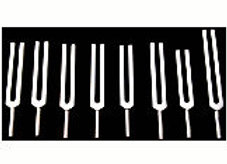# Fibonacci

SKU: diap20sp
€180.00Price
`Fibonacci are 8 tuning forks tuned to the Fibonacci numbers which are found throughout nature. In the human body, the growth of trees, shells, flowers and even the solar system you will find the Fibonacci sequence. Perfect for meditation, therapy, reiki, yoga, musical play, helps in the treatment of trauma and addictions. They are like spirals of sound between different states of consciousness.There is an exact arithmetic ratio in the forms of nature called Divine Proportion, or Phi and that is that there is a perfect ratio linking numbers, ratio and proportion including the Fibonacci series to be found for example in a daisy where you can only have 13 or 21 petals which are numbers of the Fibonacci series, count the number of leaves of an artichoke, the petals of a sunflower seeds, the arms of a starfish or the number of divisions of a fruit, you will find exactly the same proportion. A shamrock is very special and has 3 leaves and it is almost impossible to find one that has 4 leaves, which is not a Fibonacci number, the turns of the snail shell, the ellipse in which the Earth rotates, the musical scale, etc. These are all elements of nature that follow the golden ratio. This arithmetic relationship is like the "footprint of God in Nature"`